Скачать презентацию Models in I E Lecture 21 Introduction to

376f58f25970ef28788c01e0f6ef1272.ppt

• Количество слайдов: 30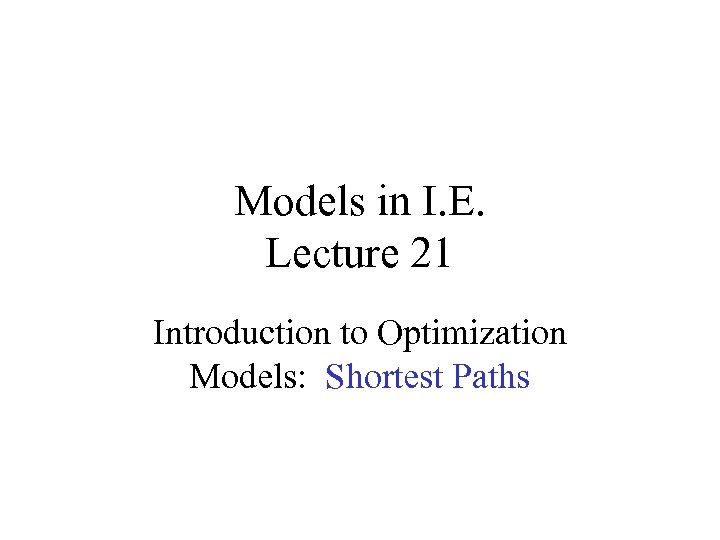Models in I. E. Lecture 21 Introduction to Optimization Models: Shortest Paths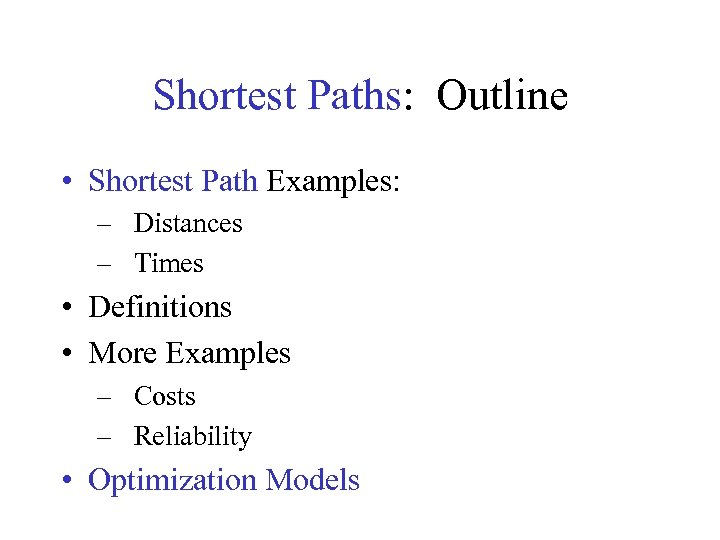Shortest Paths: Outline • Shortest Path Examples: – Distances – Times • Definitions • More Examples – Costs – Reliability • Optimization Models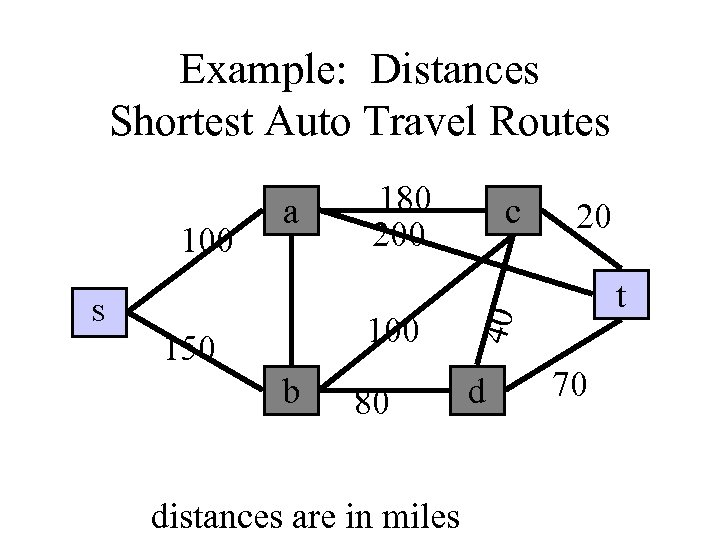Example: Distances Shortest Auto Travel Routes S 180 200 150 b 80 distances are in miles c 20 t 40 100 a d 70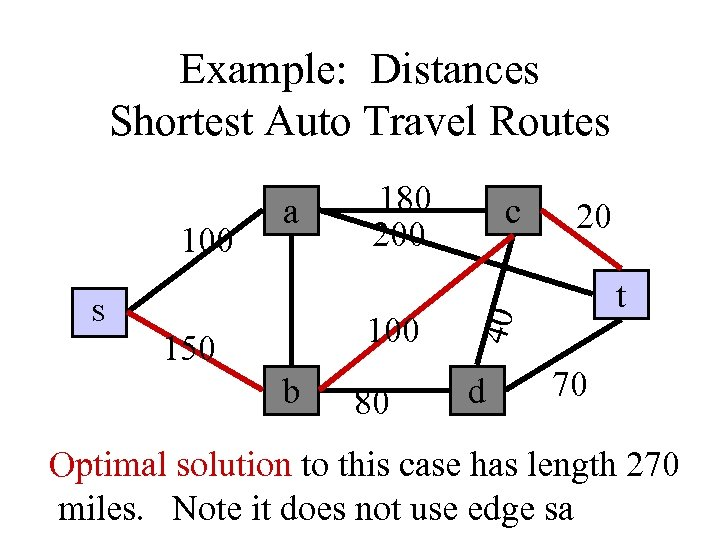Example: Distances Shortest Auto Travel Routes S 180 200 150 b 80 c 20 t 40 100 a d 70 Optimal solution to this case has length 270 miles. Note it does not use edge sa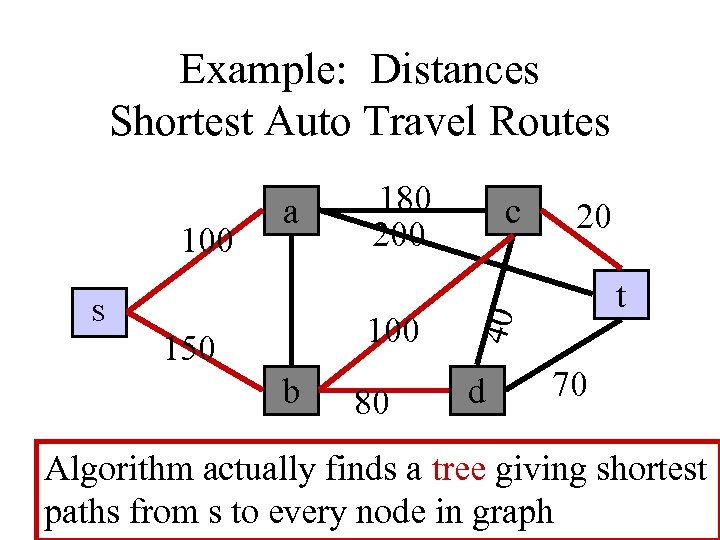Example: Distances Shortest Auto Travel Routes S 180 200 150 b 80 c 20 t 40 100 a d 70 Algorithm actually finds a tree giving shortest paths from s to every node in graph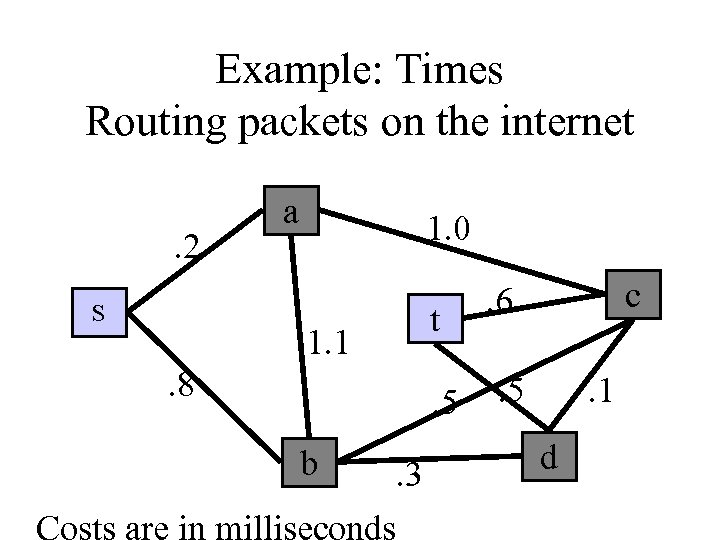Example: Times Routing packets on the internet. 2 S a 1. 0 1. 1 . 8 t. 5 b . 3 Costs are in milliseconds c . 6. 5 . 1 d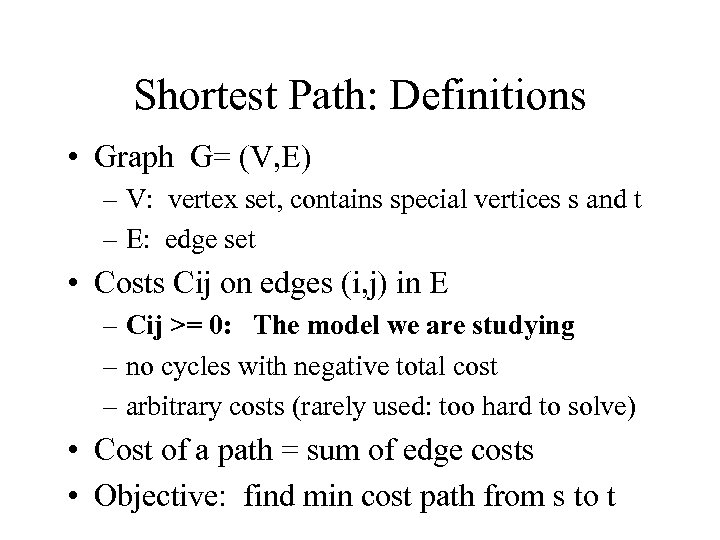Shortest Path: Definitions • Graph G= (V, E) – V: vertex set, contains special vertices s and t – E: edge set • Costs Cij on edges (i, j) in E – Cij >= 0: The model we are studying – no cycles with negative total cost – arbitrary costs (rarely used: too hard to solve) • Cost of a path = sum of edge costs • Objective: find min cost path from s to t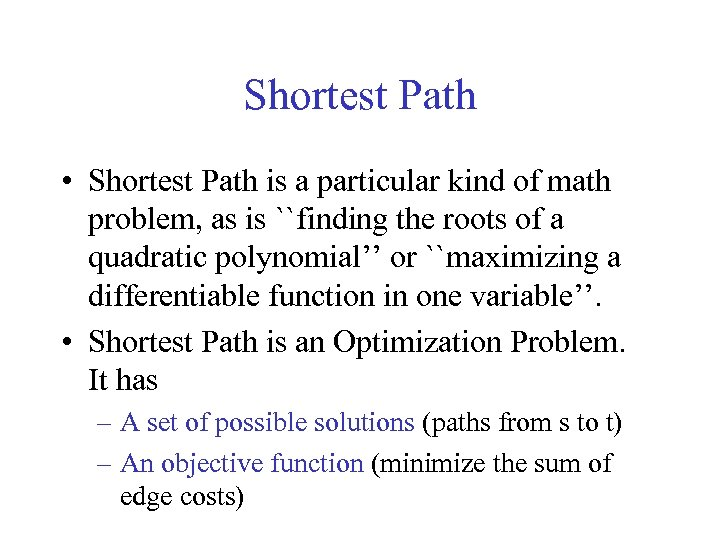Shortest Path • Shortest Path is a particular kind of math problem, as is ``finding the roots of a quadratic polynomial’’ or ``maximizing a differentiable function in one variable’’. • Shortest Path is an Optimization Problem. It has – A set of possible solutions (paths from s to t) – An objective function (minimize the sum of edge costs)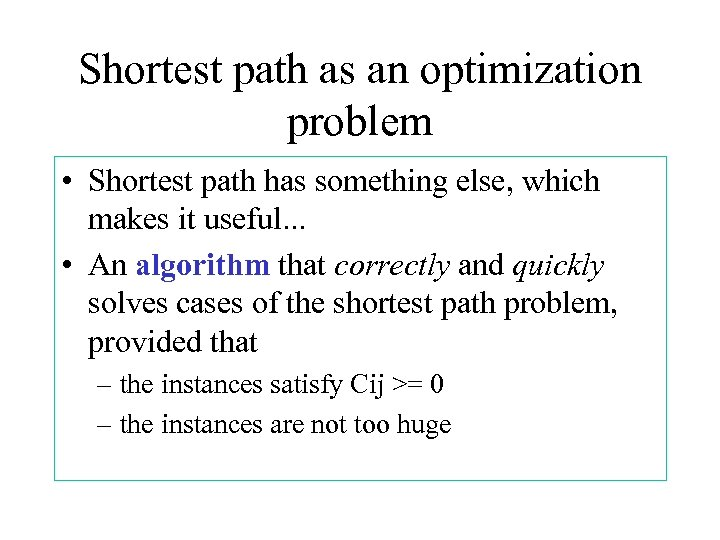Shortest path as an optimization problem • Shortest path has something else, which makes it useful. . . • An algorithm that correctly and quickly solves cases of the shortest path problem, provided that – the instances satisfy Cij >= 0 – the instances are not too huge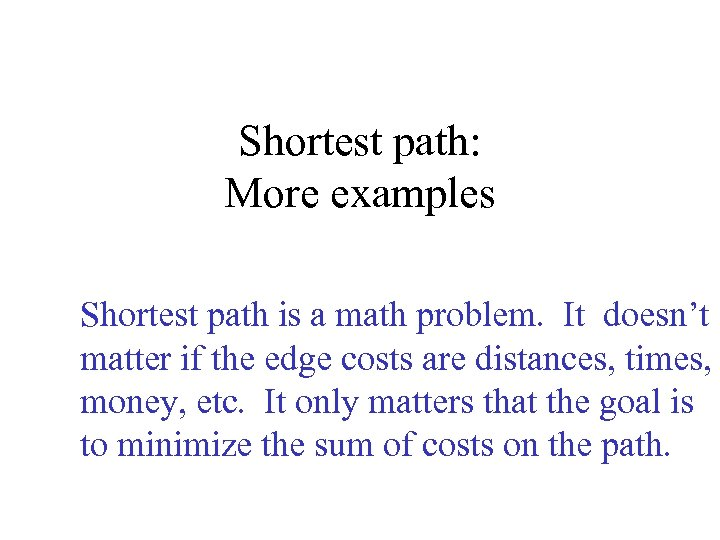Shortest path: More examples Shortest path is a math problem. It doesn’t matter if the edge costs are distances, times, money, etc. It only matters that the goal is to minimize the sum of costs on the path.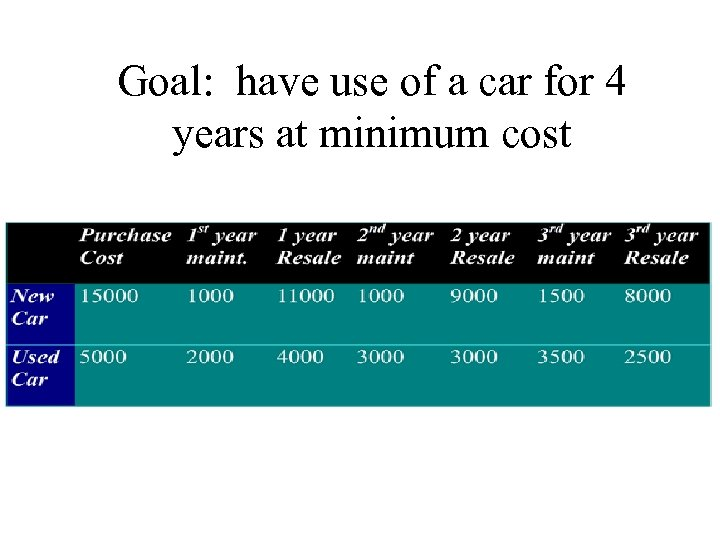Goal: have use of a car for 4 years at minimum cost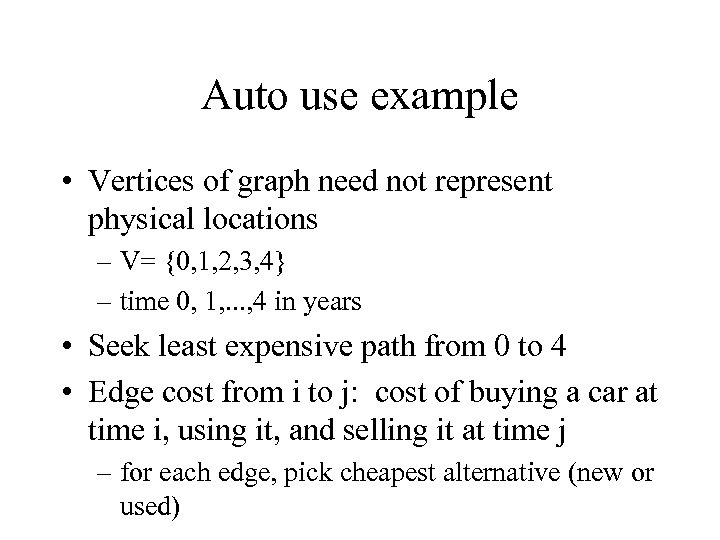Auto use example • Vertices of graph need not represent physical locations – V= {0, 1, 2, 3, 4} – time 0, 1, . . . , 4 in years • Seek least expensive path from 0 to 4 • Edge cost from i to j: cost of buying a car at time i, using it, and selling it at time j – for each edge, pick cheapest alternative (new or used)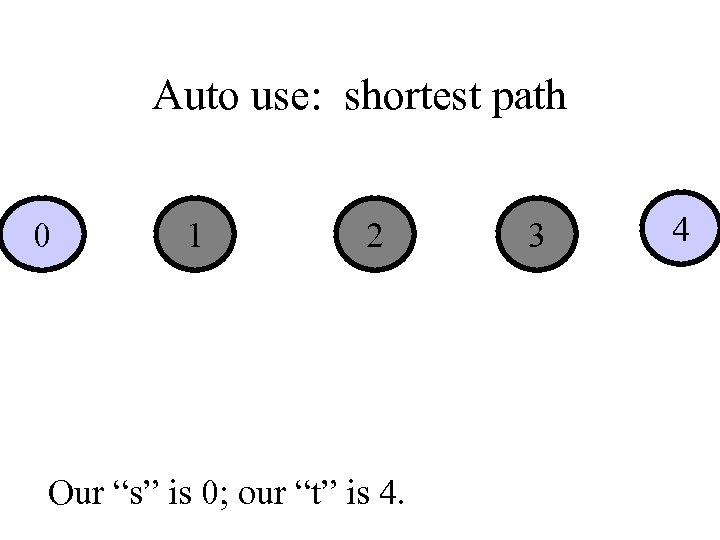Auto use: shortest path 0 1 2 Our “s” is 0; our “t” is 4. 3 4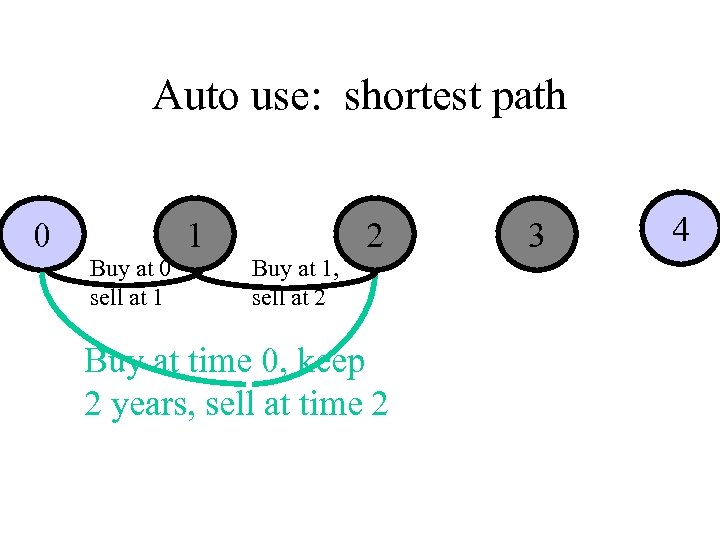Auto use: shortest path 0 Buy at 0 sell at 1 1 Buy at 1, sell at 2 2 Buy at time 0, keep 2 years, sell at time 2 3 4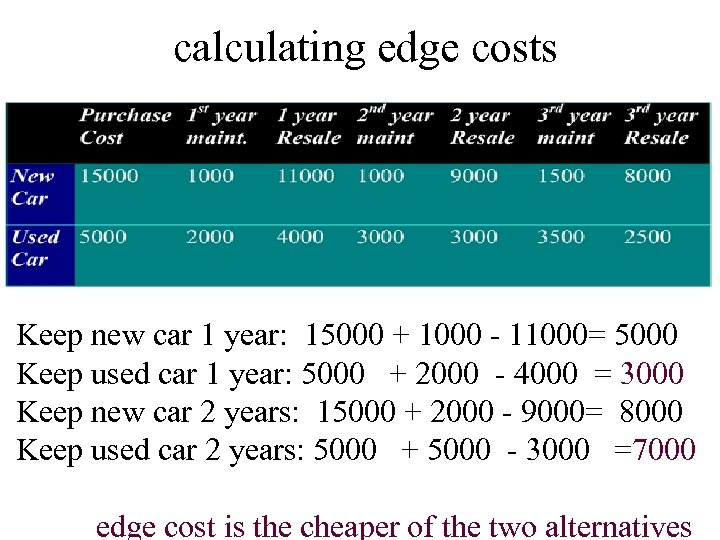calculating edge costs Keep new car 1 year: 15000 + 1000 - 11000= 5000 Keep used car 1 year: 5000 + 2000 - 4000 = 3000 Keep new car 2 years: 15000 + 2000 - 9000= 8000 Keep used car 2 years: 5000 + 5000 - 3000 =7000 edge cost is the cheaper of the two alternatives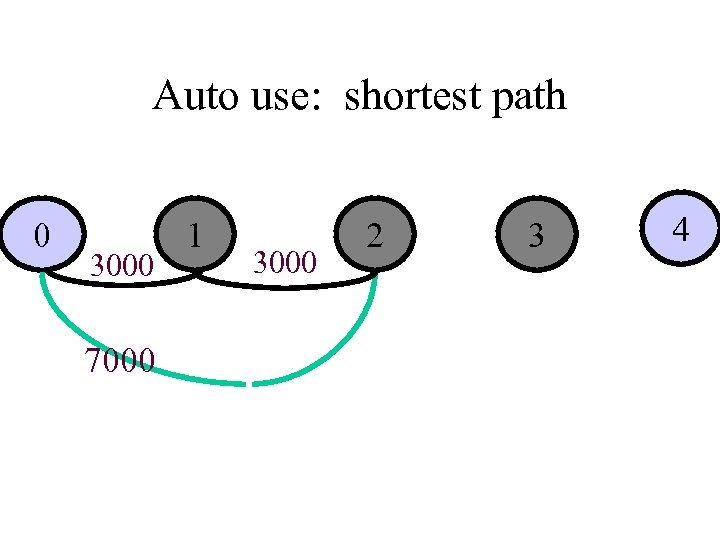Auto use: shortest path 0 3000 7000 1 3000 2 3 4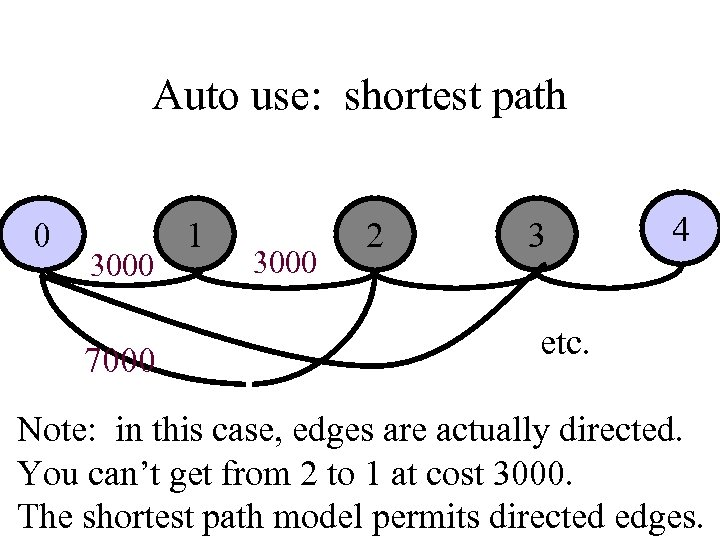Auto use: shortest path 0 3000 7000 1 3000 2 3 4 etc. Note: in this case, edges are actually directed. You can’t get from 2 to 1 at cost 3000. The shortest path model permits directed edges.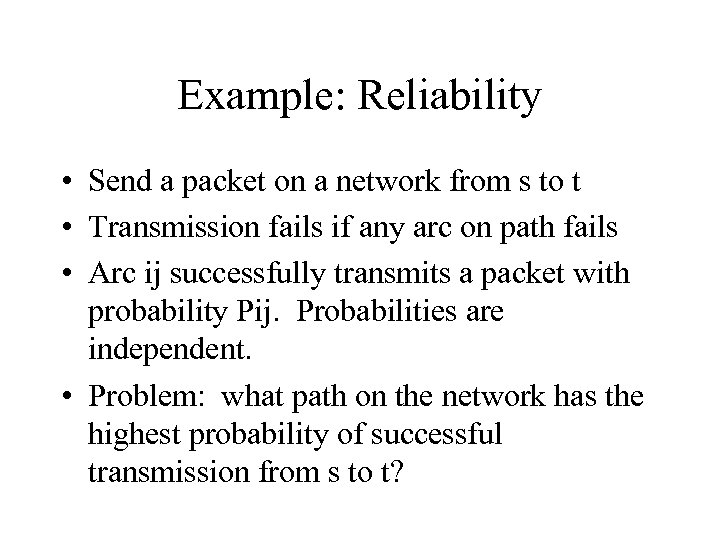Example: Reliability • Send a packet on a network from s to t • Transmission fails if any arc on path fails • Arc ij successfully transmits a packet with probability Pij. Probabilities are independent. • Problem: what path on the network has the highest probability of successful transmission from s to t?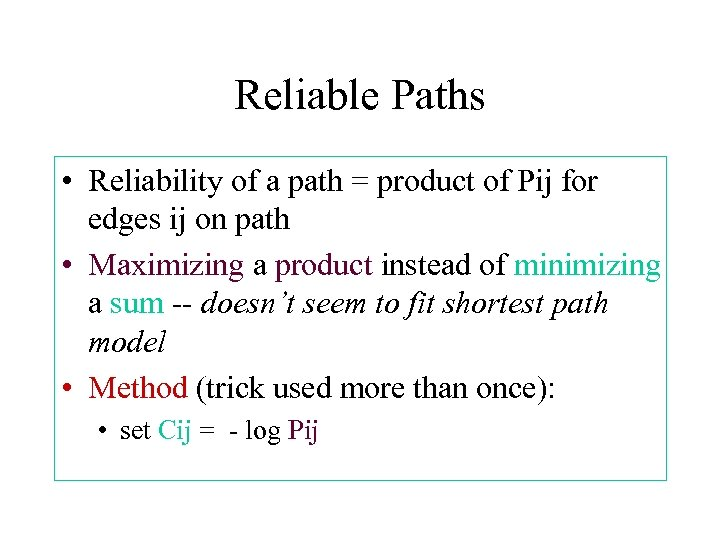Reliable Paths • Reliability of a path = product of Pij for edges ij on path • Maximizing a product instead of minimizing a sum -- doesn’t seem to fit shortest path model • Method (trick used more than once): • set Cij = - log Pij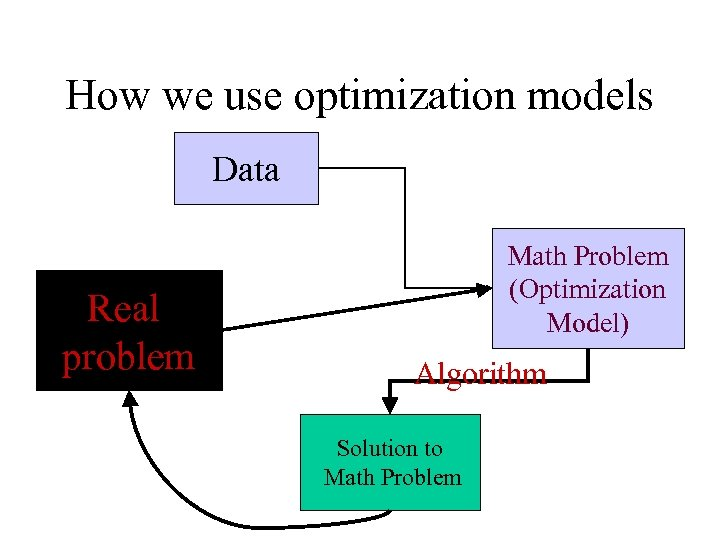How we use optimization models Data Real problem Math Problem (Optimization Model) Algorithm Solution to Math Problem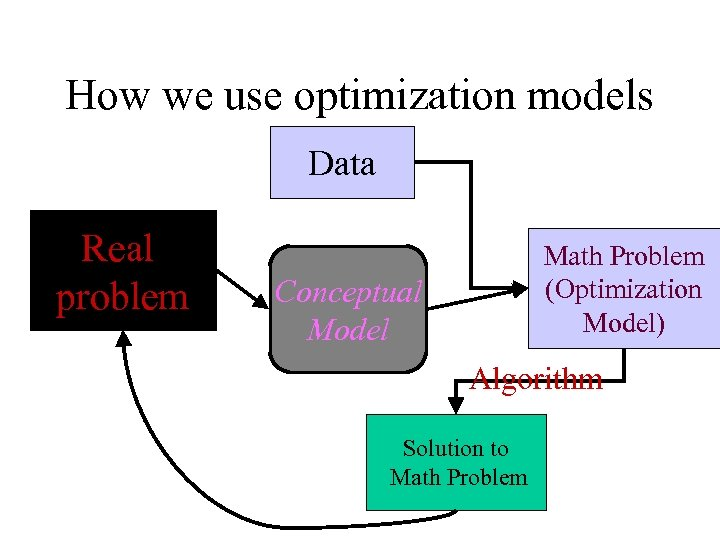How we use optimization models Data Real problem Math Problem (Optimization Model) Conceptual Model Algorithm Solution to Math Problem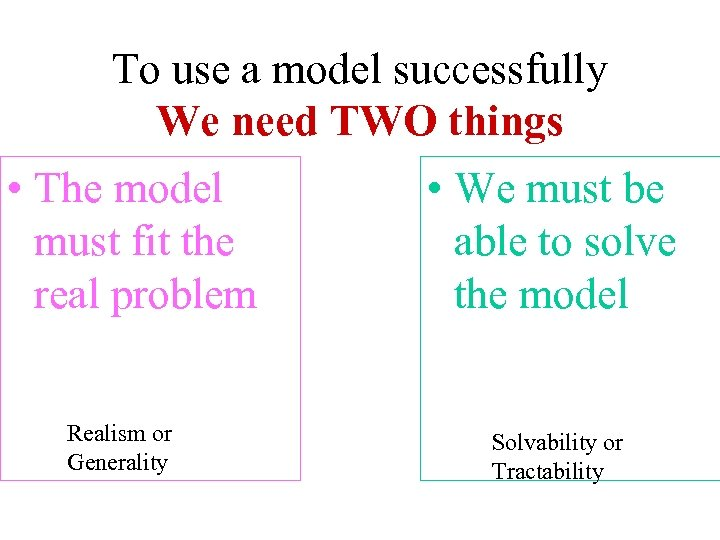To use a model successfully We need TWO things • The model must fit the real problem Realism or Generality • We must be able to solve the model Solvability or Tractability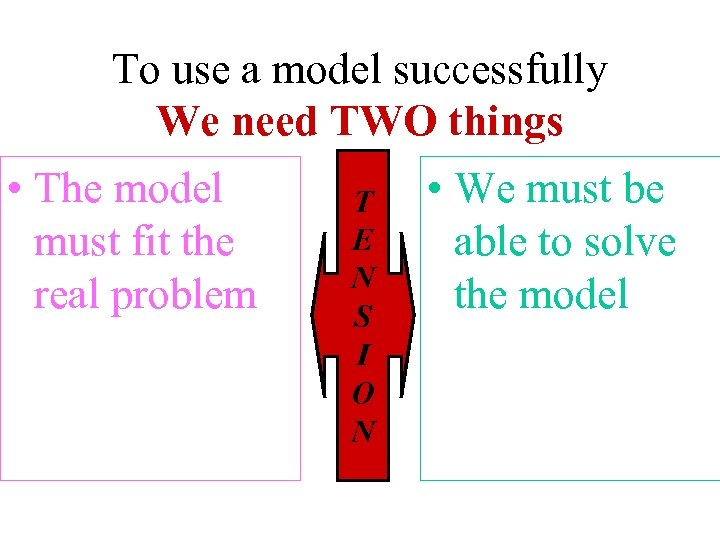To use a model successfully We need TWO things • The model must fit the real problem T E N S I O N • We must be able to solve the model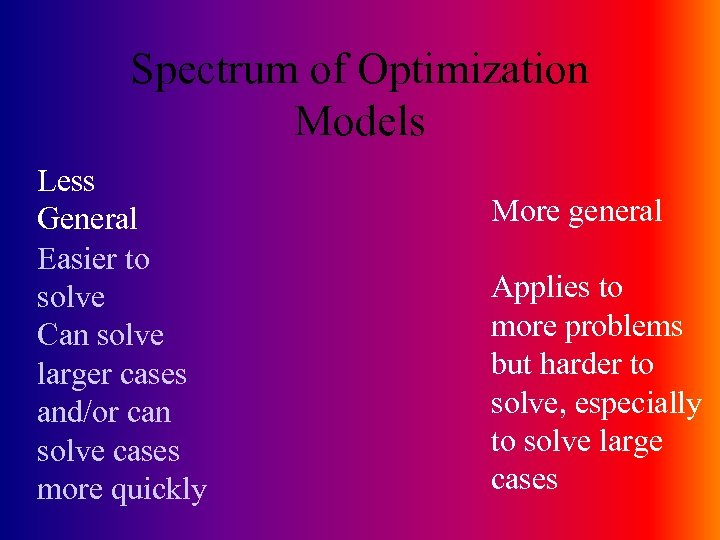Spectrum of Optimization Models Less General Easier to solve Can solve larger cases and/or can solve cases more quickly More general Applies to more problems but harder to solve, especially to solve large cases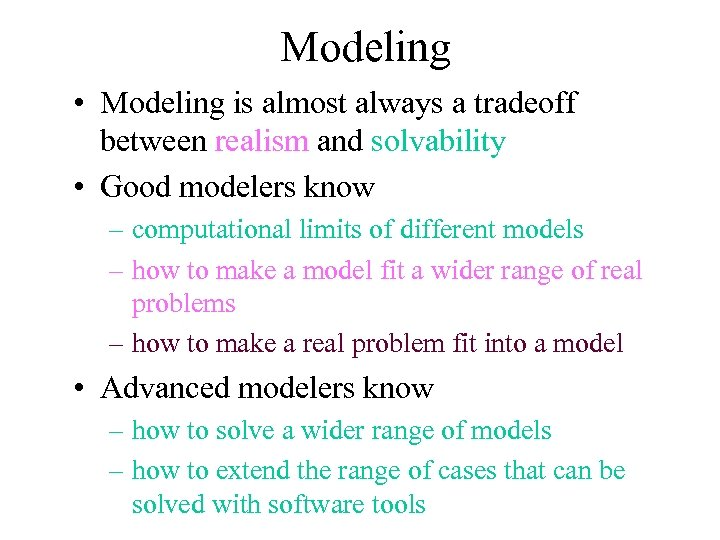Modeling • Modeling is almost always a tradeoff between realism and solvability • Good modelers know – computational limits of different models – how to make a model fit a wider range of real problems – how to make a real problem fit into a model • Advanced modelers know – how to solve a wider range of models – how to extend the range of cases that can be solved with software tools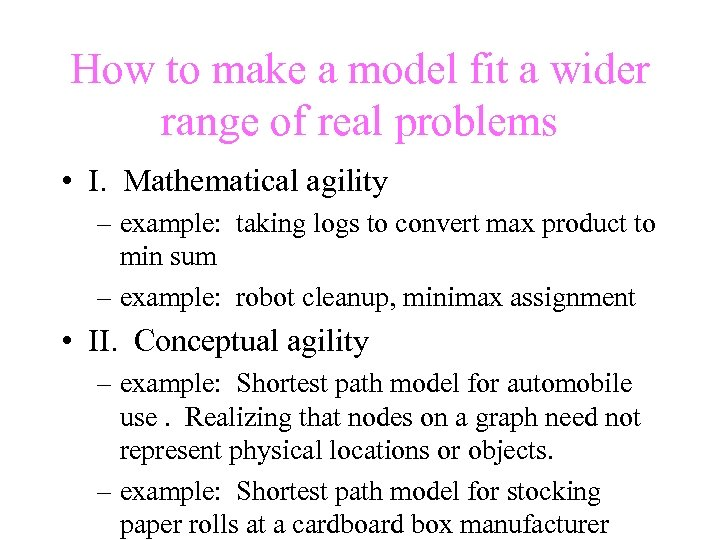How to make a model fit a wider range of real problems • I. Mathematical agility – example: taking logs to convert max product to min sum – example: robot cleanup, minimax assignment • II. Conceptual agility – example: Shortest path model for automobile use. Realizing that nodes on a graph need not represent physical locations or objects. – example: Shortest path model for stocking paper rolls at a cardboard box manufacturer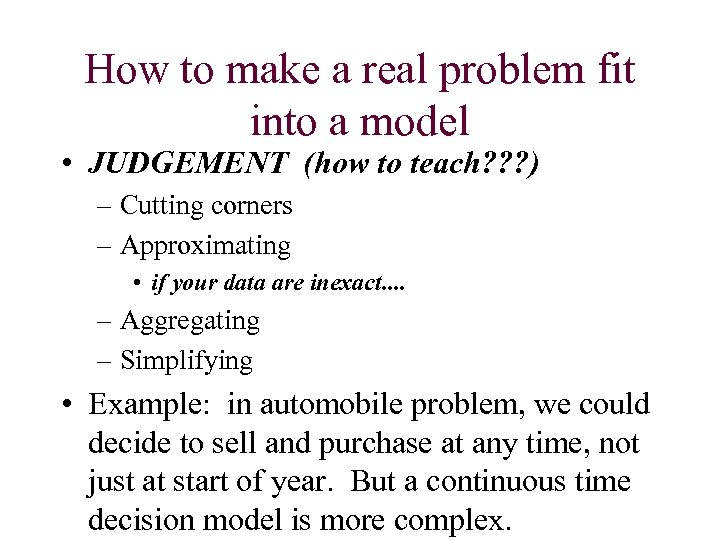How to make a real problem fit into a model • JUDGEMENT (how to teach? ? ? ) – Cutting corners – Approximating • if your data are inexact. . – Aggregating – Simplifying • Example: in automobile problem, we could decide to sell and purchase at any time, not just at start of year. But a continuous time decision model is more complex.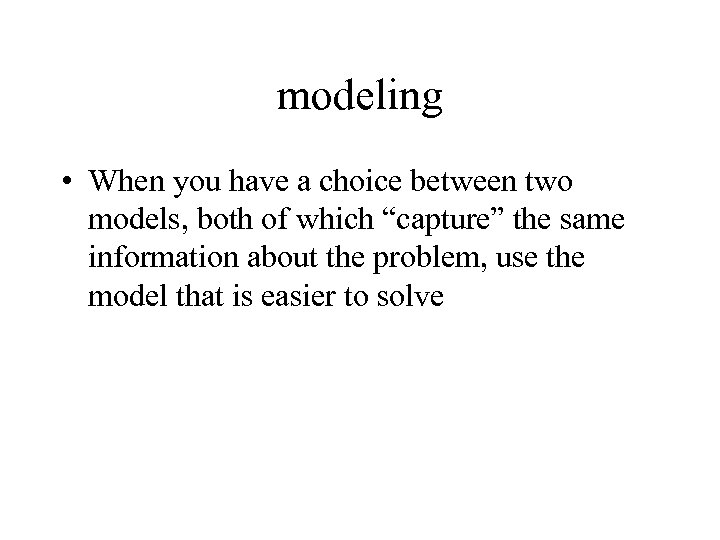modeling • When you have a choice between two models, both of which “capture” the same information about the problem, use the model that is easier to solve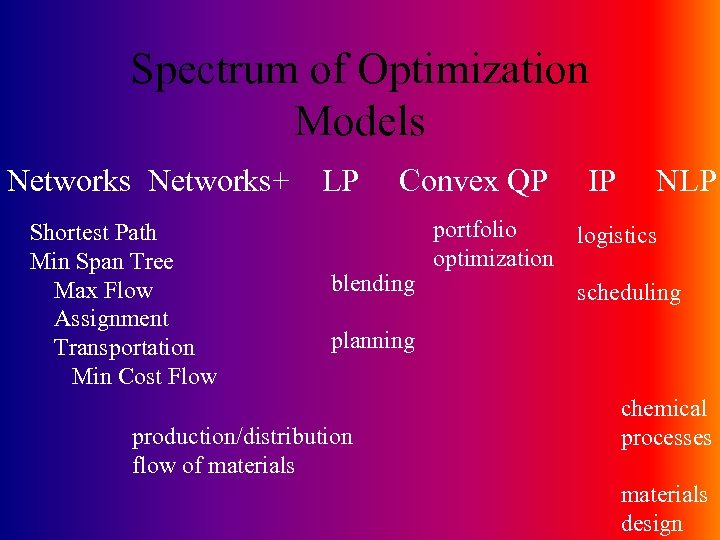Spectrum of Optimization Models Networks+ Shortest Path Min Span Tree Max Flow Assignment Transportation Min Cost Flow LP Convex QP IP NLP portfolio logistics optimization blending scheduling planning production/distribution flow of materials chemical processes materials design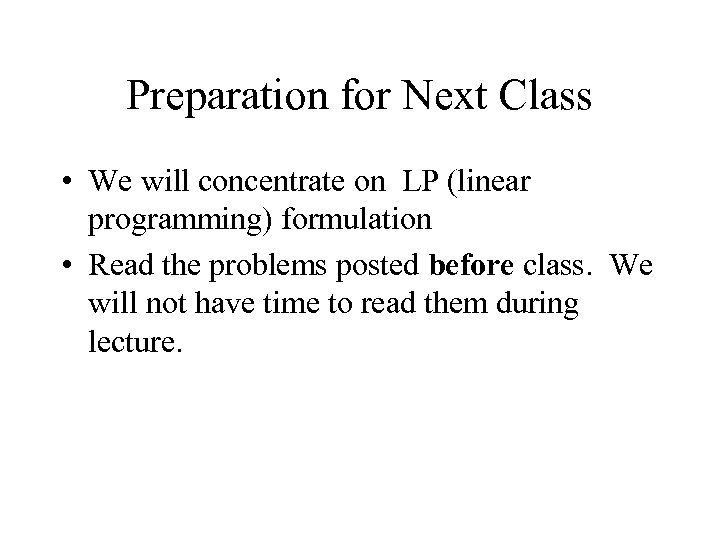Preparation for Next Class • We will concentrate on LP (linear programming) formulation • Read the problems posted before class. We will not have time to read them during lecture.